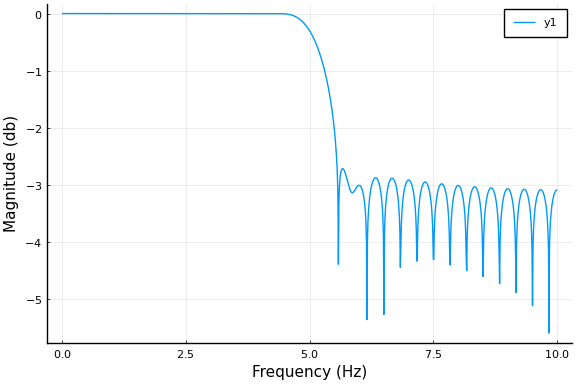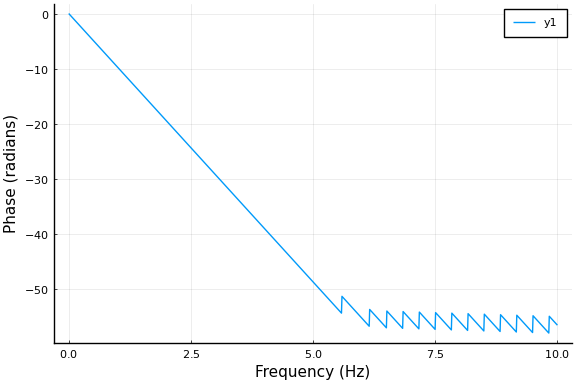Everything below the horizontal line comes from Weave. Note that Weave uses its own CSS, but there's ambiguity between the CSS that should be used by the site and Weave's (which assumes you're generating a single, standalone page). Here we copy some CSS (pertaining to the code) but you might want to do further modifications on the CSS e.g. if you want to adjust the layout (the classes used by Weave for the layout are ignored here).

Also note that Weave uses MathJax and so this page uses MathJax.

Generally speaking, if you want to use Weave, this should be a good starting point, you might have to adjust the CSS as indicated above to get a uniform experience across your site (e.g. similar code highlighting).

Note also that using Weave makes sense if you have existing notes in Weave or if you want to get some PDF output. Otherwise you'd be better off justing using Franklin, possibly in combination with Literate.

# Introduction

This an example of a julia script that can be published using Weave. The script can be executed normally using Julia or published to HTML or pdf with Weave. Text is written in markdown in lines starting with "#' " and code is executed and results are included in the published document.

Notice that you don't need to define chunk options, but you can using #+. just before code e.g. #+ term=True, caption='Fancy plots.'. If you're viewing the published version have a look at the source to see the markup.

# FIR Filter Design

We'll implement lowpass, highpass and ' bandpass FIR filters. If you want to read more about DSP I highly recommend The Scientist and Engineer's Guide to Digital Signal Processing which is freely available online.

## Calculating frequency response

DSP.jl package doesn't (yet) have a method to calculate the the frequency response of a FIR filter so we define it:

using Plots, DSP
pyplot()

function FIRfreqz(b::Array, w = range(0, stop=π, length=1024))
n = length(w)
h = Array{ComplexF32}(undef, n)
sw = 0
for i = 1:n
for j = 1:length(b)
sw += b[j]*exp(-im*w[i])^-j
end
h[i] = sw
sw = 0
end
return h
end

FIRfreqz (generic function with 2 methods)


## Design Lowpass FIR filter

Designing a lowpass FIR filter is very simple to do with DSP.jl, all you need to do is to define the window length, cut off frequency and the window. We will define a lowpass filter with cut off frequency at 5Hz for a signal sampled at 20 Hz. We will use the Hamming window, which is defined as: $w(n) = \alpha - \beta\cos\frac{2\pi n}{N-1}$, where $\alpha=0.54$ and $\beta=0.46$

fs = 20
f = digitalfilter(Lowpass(5, fs = fs), FIRWindow(hamming(61)))
w = range(0, stop=pi, length=1024)
h = FIRfreqz(f, w)

1024-element Array{Complex{Float32},1}:
1.0f0 + 0.0f0im
0.99546844f0 + 0.095055714f0im
0.98191506f0 + 0.1892486f0im
0.95946306f0 + 0.28172377f0im
0.9283168f0 + 0.37164196f0im
0.8887594f0 + 0.45818728f0im
0.84115064f0 + 0.54057467f0im
0.7859234f0 + 0.618057f0im
0.72357976f0 + 0.6899319f0im
0.65468615f0 + 0.7555481f0im
⋮
0.00043952762f0 - 0.00041908873f0im
0.0005152718f0 - 0.00040521423f0im
0.0005873293f0 - 0.00037745363f0im
0.0006531789f0 - 0.0003367371f0im
0.0007105166f0 - 0.00028444792f0im
0.0007573364f0 - 0.00022237403f0im
0.0007920005f0 - 0.00015264557f0im
0.0008132961f0 - 7.766036f-5im
0.0008204784f0 - 3.1148685f-18im


## Plot the frequency and impulse response

The next code chunk is executed in term mode, see the script for syntax.

julia> h_db = log10.(abs.(h));

julia> ws = w/pi*(fs/2)
0.0:0.009775171065493646:10.0

plot(ws, h_db,
xlabel = "Frequency (Hz)", ylabel = "Magnitude (db)")And again with default options

h_phase = unwrap(-atan.(imag.(h),real.(h)))
plot(ws, h_phase,
xlabel = "Frequency (Hz)", ylabel = "Phase (radians)")